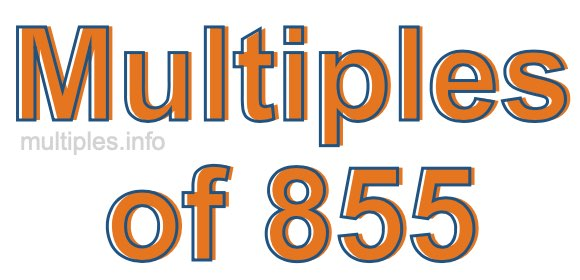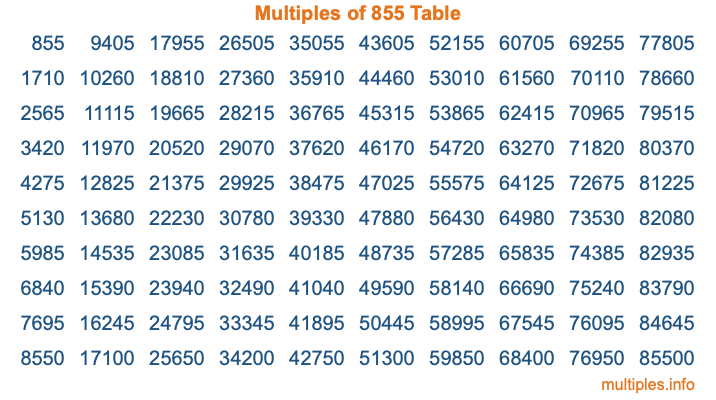Multiples of 855Welcome to the Multiples of 855 page. Here we will first teach you everything you will ever need to know about the multiples of 855, and then give you a study guide summary of everything we taught you to make sure you remember it all. Use this page to look up facts and learn information about the multiples of 855. This page will make you a multiples of eight hundred fifty-five expert!

Definition of Multiples of 855
Multiples of 855 are all the numbers that when divided by 855 equal an integer. Each of the multiples of 855 are called a multiple. A multiple of 855 is created by multiplying 855 by an integer.

Therefore, to create a list of multiples of 855, you start with 1 multiplied by 855, then 2 multiplied by 855, then 3 multiplied by 855, and so on for as long as you want. Thus, the list of the first five multiples of 855 is 855, 1710, 2565, 3420, and 4275. To see a larger list of multiples of 855, see the printable image of Multiples of 855 further down on this page. We also have a category where you can choose any nth multiple of 855.

Multiples of 855 Checker
The Multiples of 855 Checker below checks to see if any number of your choice is a multiple of 855. In other words, it checks to see if there is any number (integer) that when multiplied by 855 will equal your number. To do that, we divide your number by 855. If the the quotient is an integer, then your number is a multiple of 855.

Is  a multiple of 855?

Least Common Multiple of 855 and ...
A Least Common Multiple (LCM) is the lowest multiple that two or more numbers have in common. This is also called the smallest common multiple or lowest common multiple and is useful to know when you are adding our subtracting fractions. Enter one or more numbers below (855 is already entered) to find the LCM.

Check out our LCM Calculator if you need more details about the Least Common Multiple or if you need the LCM for different numbers for adding and subtraction fractions.

nth Multiple of 855
As we stated above, 855 is the first multiple of 855, 1710 is the second multiple of 855, 2565 is the third multiple of 855, and so on. Enter a number below to find the nth multiple of 855.

th multiple of 855

Multiples of 855 vs Factors of 855
855 is a multiple of 855 and a factor of 855, but that is where the similarities end. All postive multiples of 855 are 855 or greater than 855. All positive factors of 855 are 855 or less than 855.

Below is the beginning list of multiples of 855 and the factors of 855 so you can compare:

Multiples of 855: 855, 1710, 2565, 3420, 4275, etc.

Factors of 855: 1, 3, 5, 9, 15, 19, 45, 57, 95, 171, 285, 855

As you can see, the multiples of 855 are all the numbers that you can divide by 855 to get a whole number. The factors of 855, on the other hand, are all the whole numbers that you can multiply by another whole number to get 855.

It's also interesting to note that if a number (x) is a factor of 855, then 855 will also be a multiple of that number (x).

Multiples of 855 vs Divisors of 855
The divisors of 855 are all the integers that 855 can be divided by evenly. Below is a list of the divisors of 855.

Divisors of 855: 1, 3, 5, 9, 15, 19, 45, 57, 95, 171, 285, 855

The interesting thing to note here is that if you take any multiple of 855 and divide it by a divisor of 855, you will see that the quotient is an integer.

Multiples of 855 Table
Below is an image of the first 100 multiples of 855 in a table. The table is in chronological order, column by column. The first column has the first ten multiples of 855, the second column has the next ten multiples of 855, and so on.The Multiples of 855 Table is also referred to as the 855 Times Table or Times Table of 855. You are welcome to print out our table for your studies.

Negative Multiples of 855
Although not often discussed or needed in math, it is worth mentioning that you can make a list of negative multiples of 855 by multiplying 855 by -1, then by -2, then by -3, and so on, to get the following list of negative multiples of 855:

-855, -1710, -2565, -3420, -4275, etc.

Multiples of 855 Summary
Below is a summary of important Multiples of 855 facts that we have discussed on this page. To retain the knowledge on this page, we recommend that you read through the summary and explain to yourself or a study partner why they hold true.

There are an infinite number of multiples of 855.

A multiple of 855 divided by 855 will equal a whole number.

855 divided by a factor of 855 equals a divisor of 855.

The nth multiple of 855 is n times 855.

The largest factor of 855 is equal to the first positive multiple of 855.

855 is a multiple of every factor of 855.

855 is a multiple of 855.

A multiple of 855 divided by a divisor of 855 equals an integer.

855 divided by a divisor of 855 equals a factor of 855.

Any integer times 855 will equal a multiple of 855.

Multiples of a Number
Here you can get the multiples of another number, all with the same attention to detail as we did for multiples of 855 on this page.

Multiples of
Multiples of 856
Did you find our page about multiples of eight hundred fifty-five educational? Do you want more knowledge? Check out the multiples of the next number on our list!